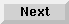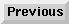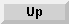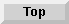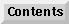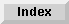4 Data Variables and Data handling

## XPACK, XUNPACK statements

The XPACK and XUNPACK statements provide a convenient way to transfer string and numeric data to and from a string variable. They are particular useful in conjunction with Dialog Manager record objects.

Unlike the PACK USING and UNPACK USING statements, the XPACK and XUNPACK statements include the variable name in the packed string and use a variable length string format.

### The XPACK statement

The XPACK statement transfers data from each variable of the variable list to the destination string. A variable list may be specified in three different ways:

• a string expression evaluating in a variable list

• a variable list

• a reference to IN DATA SET LIST variable lists

A reference to a whole array will be resolved into a list of array elements.

As the data is transferred to the destination string, it is converted into string format and stored along with the variable name and array index.

The XPACK and XUNPACK statements can operate on a STRUCT. STRUCT is treated as a list of variables.

The XPACK statement packs each member variable in a buffer. The XUNPACK statement unpacks to a user defined type if it is passed as an argument to XUNPACK and the member variable matches the name in the buffer.

#### XPACK ... USING

XPACK Dest\$ USING <string expression>

Pack destination string variable from variable list specified by the string expression. The string expression contains a list of variable names separated by a comma.

For example:

```   List\$="A,A\$,B,B\$,A\$(1),Array\$(*)"
```

#### XPACK ... USING REMOTE LISTS

XPACK Dest\$ USING REMOTE LISTS Label [ ,Label . . . ]

Pack destination string variable from variable list as defined by the referenced remote list(s).

For example:

```   XPACK Dest\$ USING REMOTE LISTS Label1,Label2
Label1: IN DATA SET LIST A,A\$
Label2: IN DATA SET LIST ...
```

#### XPACK ... FROM

XPACK Dest\$ FROM Variable [ ,Variable . . . ]

Pack destination string variable from variable list.

For example:

```   XPACK Dest\$ FROM A,A\$,B,B\$,A\$(*)
```

### The XUNPACK statement

The XUNPACK statement transfers data from a string variable into the original variables. If a variable list is specified, only those variables are unpacked, that are included in the variable list. It's not possible to unpack a buffer into a different variable than used to pack the buffer.

XUNPACK Buf\$

Unpack buffer variable into variables as named in the buffer.

For example:

```   A\$="Test"
B=123
XPACK Buf\$ FROM A\$,B
A\$=""
B=0
XUNPACK Buf\$
```
This recovers the initial values of A\$ and B.

#### XUNPACK Buf\$ USING <string expression>

Unpack buffer variable into variables as named in the buffer. The string expression contains a list of variable names separated by a comma. Only variables included in the variable list are unpacked.

For example:

```   XPACK Buf\$ FROM A\$,B,C
List\$="A\$,B"
XUNPACK Buf\$ USING List\$
```
This should unpack the variables A\$ and B only.

#### XUNPACK Buf\$ FROM Variable [,Variable ...]

Unpack buffer variable into variables as named in the buffer. Only variables included in the variable list are unpacked.

For example:

```   XPACK Buf\$ FROM A\$,B,C
XUNPACK Buf\$ FROM A\$,B
```
This unpacks the variables A\$ and B only.

#### XUNPACK Buf\$ USING REMOTE LISTS Line_id[,Line_id ...]

Unpack buffer variable into variables as named in the buffer. Only variables included in the referenced REMOTE LISTS list are unpacked.

For example:

```   XPACK Buf\$ FROM A\$,B,C
XUNPACK Buf\$ USING REMOTE LISTS Label
Label: IN DATA SET LIST A\$,B
```

### XPACK format description

Each variable in a XPACK statement is converted into a string format containing fields describing variable type, name, index and value.

The following rules apply:

• Format fields are separated by tilde characters ('~').

• All fields are printable (no binary data).

• Numeric values are converted into string.

 Type Name ~ Idx ~ Len ~ Value ... \$

~
Field separator. The Tilde character ('~').
Type
Field type. One of the following:
N

numeric

X

string

\$

End-of-list

Name
Name = field name. Up to 15 characters. Must be valid Variable name. Trailing \$ of string variables is omitted.
Idx
Array index. 0 = simple variable.
First array element is 1 (independent of array bounds and number of dimensions).
Len
Size (number of bytes) of value field.
Value
Value field. Any number of characters.

#### Simple numeric variable

```Simple = 47
XPACK Buf\$ FROM Simple
```
Results in the following buffer:

```   "NSimple~0~2~47\$"
```
Equivalent Eloquence code:

```Buf\$="N"&"Simple~"&"0~"&VAL\$(LEN(VAL\$(Simple)))
&"~"&VAL\$(Simple)&"\$"
```

#### Simple string variable

```Simple\$ = "String"
XPACK Buf\$ FROM Simple\$
```
Results in the following buffer:

```   "XSimple~0~6~String\$"
```
Equivalent Eloquence code:

```Buf\$="X"&"Simple~"&"0~"&VAL\$(LEN(Simple\$))&"~"&Simple\$&"\$"
```

#### Array element

```A\$(1)="TEST"
XPACK Buf\$ USING "A\$(1)"
```
Results in the following buffer:

```   "XA~1~4~TEST\$"
```

#### Array

```DIM A\$(0:3)
A\$(0)="000"
A\$(1)="111"
A\$(2)="222"
A\$(3)="333"
XPACK Buf\$ FROM A\$(*)
```
Results in the following buffer:

```"XA~1~3~000XA~2~3~111XA~3~3~222XA~4~3~333\$"
```

#### Example Eloquence XUNPACK program

The example program below shows how to unpack a buffer in XPACK format. This example has been provided only for clarification of XPACK format.

```DIM Type\$,Var_name\$,Value\$,Variable\$
INTEGER P,Idx,Len

LOOP
Type\$=Buf\$[1,1]            ! Type
EXIT IF Type\$="\$"
Buf\$=Buf\$
P=POS(Buf\$,"~")            ! locate separator
Var_name\$=Buf\$[1;P-1]      ! Variable name
Buf\$=Buf\$[P+1]
P=POS(Buf\$,"~")            ! locate separator
Idx=VAL\$(Buf\$[1,P-1])      ! Array index
Buf\$=Buf\$[P+1]
P=POS(Buf\$[P],"~")         ! Separator
Len=VAL(Buf\$[1,P-1])       ! Value length
Buf\$=Buf\$[P+1]
Value\$=Buf\$[1;Len]         ! Value
Buf\$=Buf\$[Len+1]
IF Idx THEN
Variable\$=Var_name\$&"("&VAL\$(Idx)&")"
ELSE
Variable\$=Var_name\$
END IF
IF Type\$="X" THEN
COMMAND Variable\$&"\$=Value\$"
ELSE
COMMAND Variable\$&"="&Value\$
END IF
END LOOP
```

Eloquence Language Manual - 19 DEC 2002